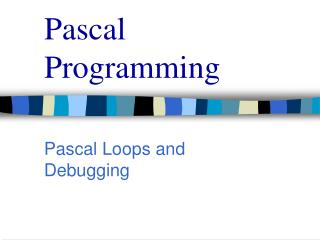# Pascal Programming - PowerPoint PPT PresentationDownload PresentationPascal Programming

Pascal Programming
Download Presentation## Pascal Programming

- - - - - - - - - - - - - - - - - - - - - - - - - - - E N D - - - - - - - - - - - - - - - - - - - - - - - - - - -
##### Presentation Transcript

1. Pascal Programming Pascal Loops and Debugging

2. Pascal Programming • Pascal Loops • In our first brush with the while do loops, simple comparisons were used. • Now, we add the Boolean expression. • As long as the expression evaluates as true, the loop continues. False stops the looping.

3. Pascal Programming • The repeat statement, another method of looping. • Syntax . . . • repeat • Statement or statements; • until • Boolean-expression • end

4. Pascal Programming • The body of a repeat may contain multiple statements . . . • The body of the while statement contains one statement. • The difference holds limited significance because the while statement may be compound.

5. Pascal Programming • The for loop . . . • Syntax . . . • For Control_variable := Initial_expression to Final_expression do • Body • The value increments by one each time through the loop. • The body of the for loop can not change the loop-control variable. • The loop-control variable is intended for exclusive use of the loop. (a local variable)

6. Pascal Programming • The second version of the for loop decrements . . . • In the statement downto replaces to • Downto is a reserved word. • Each time through the loop it decreases by one. • Note: Pascal only increments by one or decrements by one. You must design around this limitation. • e g. You want 0, 2, 4, 6, 8, 10 . . . • For N := 0 to 5 do • Write (2*N)

7. Pascal Programming • When designing a Pascal loop, design… • The Body • The initializing statements • The conditions for exiting the loop. • We have talked about the first two no let’s look at terminating the loop.

8. Pascal Programming • Terminating a loop . . . • Terminating input • List is headed by size (list size is known) • Ask before iterating (ask the user) • List ended by sentinal value. • Input runs out.

9. Pascal Programming • Terminating the loop . . . • Count the controlled loops. • Ask before iterating. • Exit on sentinel value. • Input runs out. • Exit on a flag condition. • A flag is a variable that changes value to indicate that an event has occurred. • The end of line (eoln) and end of file (eof) are examples. (Eoln does not work well with numeric data. It prefers char type.)

10. Pascal Programming • What loop should I use? • Numeric data, fixed iterations, equal changes each time . . .use for • Generally, the for loop will be best for numeric data. • If the loop may not be used at least once, use while. It also works when no data may be entered from a list. • If you insist that the loop be used at least once, use repeat.

11. Pascal Programming • Assertions • Comments about pre- and post- conditions are called assertions. • An invariant assertion is true before the loop runs and true after. The variable changes with each iteration but holds true with each iteration. Thus it is invariant. continue . . .

12. Pascal Programming • Assertions cont . . . • A variant assertion changes after each iteration. • Two conditions . . . • The value must decrease by a fixed amount (or more) each iteration. • When the variant equals or falls below the threshold, the loop must stop. • The threshold is the point to be reached or passed.

13. Pascal Programming • Debugging Loops • For repeat or while loops . . .check the Boolean expression. Is something reversed? • Be sure that the error is in the loop. • Trace the data through the loop. • Check intervals in long loops.